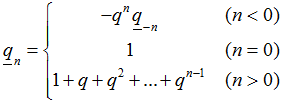# Some properties of Fermatian numbers

A. G. Shannon
Notes on Number Theory and Discrete Mathematics, ISSN 1310-5132
Volume 10, 2004, Number 2, Pages 25—33
Download full paper: PDF, 152 Kb

## Details

### Authors and affiliations

A. G. Shannon
Warrane College, The University of New South Wales, Kensington 1465, &
KvB Institute of Technology, 99 Mount Street, North Sydney, NSW 2065, Australia

### Abstract

This paper looks at some basic number theoretic properties of Fermatian numbers. We define the n-th reduced Fermatian number in terms ofso that 1n = n, and 1n! = n!, where qn! = qnqn−1q1.
Some congruence properties and relationships with Bernoulli and Fibonacci numbers are explored. Some aspects of the notation and meaning of the Fermatian numbers are also outlined.

• 11B65
• 11B39
• 05A30

### References

1. L. Carlitz. A Set of Polynomials. Duke Mathematical Journal. 6 (1940): 486-504.
2. L. Carlitz. q-Bernoulli Numbers and Polynomials. Duke Mathematical Journal. 15 (1948): 987-1000.
3. L. Carlitz. A Theorem of Glaisher. Canadian Journal of Mathematics. 5 (1953): 306-316.
4. L. Carlitz. Note on a Theorem of Glaisher.  Journal of the London Mathematical Society. 28 (1953): 245-246.
5. L. Carlitz. Extension of a Theorem of Glaisher and Some Related Results. Bulletin of the Calcutta Mathematical Society. 46 (1954): 77-80.
6. L. Carlitz. A Note on the Generalized Wilson’s Theorem. American Mathematical Monthly. 71 (1964): 291-293.
7. L. Carlitz. The Highest Power of a Prime Dividing Certain Quotients. Archiv der Mathematik. 18 (1967): 153-159.
8. L. Carlitz. Some Identities in Combinatorial Analysis. Duke Mathematical Journal 38 (1971): 51-56.
9. L. Carlitz & L. Moser. On Some Special Factorizations of . Canadian Mathematical Bulletin. 9 (1966): 421-426.
10. A. L. Cauchy. Memoire sur les functions don’t plusiers valeurs. Comptes rendus de l’Académie des Sciences. 17 (1843): 526-534.
11. N. Cox, J. W. Phillips & V.E. Hoggatt Jr. Some Universal Counterexamples. The Fibonacci Quarterly. (1970): 242-248.
12. L. E. Dickson. History of the Theory of Numbers.Volume 1.  NewYork: Chelsea, 1952.
13. M. Feinberg. Fibonacci-tribonacci.The Fibonacci Quarterly. 1(3) (1963): 71-74.
14. M. Feinberg. New Slants. The Fibonacci Quarterly. 2(1964): 223-227.
15. G. Fontené. Generalization d’une formule connue. Nouvelles Annales Mathématiques. 15 (1915): 112.
16. H. W. Gould. The Bracket Function and Fontené-Ward Generalized Binomial Coefficients with Application to Fibonacci Coefficients. The Fibonacci Quarterly. 7 (1969): 23-40,55.
17. G. H. Hardy & E. M. Wright. An Introduction to the Theory of Numbers. Oxford: The Clarendon Press, 1965.
18. V. E. Hoggatt Jr & M. Bicknell. Diagonal Sums of Generalized Pascal Triangles. The Fibonacci Quarterly. 7 (1969): 341-358.
19. A. F. Horadam. Generating Functions for Powers of a Certain Generalized Sequence of Numbers. Duke Mathematical Journal. 32 (1965): 437-446.
20. A. F. Horadam & A. G. Shannon. Ward’s Staudt-Clausen Problem. Mathemetica Scandinavica. 29 (1976): 239-250.
21. P. A. Macmahon. Combinatory Analysis. Cambridge: Cambridge University Press, 1916.
22. J. Riordan. An Introduction to Combinatorial Analysis. New York: Wiley, 1958, p.3.
23. J. Riordan. A Note on a q-extension of Ballot Numbers. Journal of Combinatorial Theory. 4 (1968): 191-193.
24. A. G. Shannon, Some Fermatian Special Functions, Notes on Number Theory & Discrete Mathematics, in press.
25. G. J. Tee. Russian Peasant Multiplication and Egyptian Division in Zeckendorf Arithmetic. Australian Mathematical Society Gazette. 30 (2003): 267-276.
26. M. Ward. A Calculus of Sequences. American Journal of Mathematics. 75 (1936): 255-266.

## Cite this paper

APA

Shannon, A. G. (2004). Some properties of Fermatian numbers. Notes on Number Theory and Discrete Mathematics, 10(2), 25-33.

Chicago

Shannon, A. G. “Some Properties of Fermatian Numbers.” Notes on Number Theory and Discrete Mathematics 10, no. 2 (2004): 25-33.

MLA

Shannon, A. G. “Some Properties of Fermatian Numbers.” Notes on Number Theory and Discrete Mathematics 10.2 (2004): 25-33. Print.

Comments are closed.